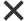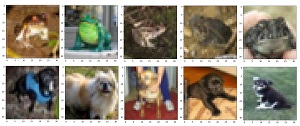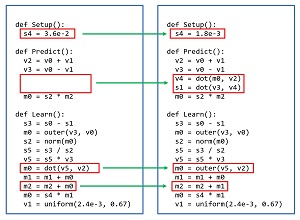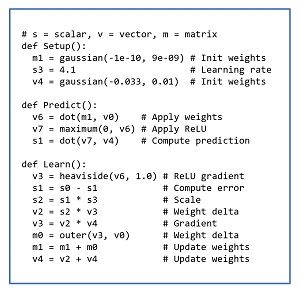### The AutoML-Zero System Automatically Generates Machine Learning Programs

The Pure AI editors explain a new paper that describes how a computer program can automatically generate a machine learning algorithm, which can create a machine learning prediction model.In the 1973 movie "Westworld," rich people go to a futuristic amusement park that is populated by perfect replica robots. Well, perfect until the robots start running amok and killing the guests. In the control center, the operations manager asks the Chief Supervisor what is going wrong. The Chief Supervisor replies, "We aren't dealing with ordinary machines here. These are highly complicated pieces of equipment. Almost as complicated as living organisms. In some cases, they have been designed by other computers. We don't know exactly how they work."

Machine learning (ML) researchers from Google have published a paper titled "AutoML-Zero: Evolving Machine Learning Algorithms from Scratch" which describes how a computer program can automatically generate a machine learning algorithm, which can create a machine learning prediction model. The AutoML-Zero system uses a form of evolutionary optimization. Starting from a set of empty computer programs that do nothing, over time a sophisticated ML image classification algorithm evolves that can match or sometimes even exceed the classification accuracy of some complex, hand-coded algorithms.

Remarkably, the algorithms automatically generated by AutoML-Zero include algorithms that are the result of decades of human ML research such as perceptron learning and neural networks. And when seeded with a basic neural framework, AutoML-Zero discovered training optimization techniques such as regularization, weight decay, and adaptive learning rate.

General Evolutionary Optimization
Evolutionary optimization (EO) is a meta-heuristic (set of general guidelines) that can be used to construct a specific algorithm that solves a problem. EO guidelines are very loosely based on ideas related to processes found in biological systems, such as natural selection and chromosome mutation. EO is one of many bio-inspired meta-heuristics. Other bio-inspired techniques include genetic algorithm numerical optimization, simulated bee colony combinatorial optimization, and ant colony graph search optimization.

General EO is best understood by an example. Suppose you want to find the values of x and y that minimize the function f(x,y) = x^2 + y^2. The solution is x = 0.0 and y = 0.0, which could be easily found using standard techniques from mathematical Calculus. In pseudo-code, one possible EO algorithm for solving the problem is:

```create a set of 10 random, possible solutions
loop 1000 times
evaluate goodness of each possible solution
pick two good solutions as parents
use parents to create a new child solution
mildly mutate the child solution
replace a poor solution with child solution
end-loop
return best solution found

```

For this simple numerical optimization example, each possible solution is a vector with two values, such as (2.5, -1.3). The goodness of a possible solution could be measured by evaluating the objective function using the solution, where a smaller value of f(x, y) is better.

General AutoML
The term AutoML is used to describe any system that programmatically, to some extent, determines or computes part of an ML prediction system with limited or no human input. One example is programmatically finding a good architecture for a neural network classifier, including the number of hidden layers, number of nodes in each hidden layer, hidden layer activation function, and so on. Another example is hyperparameter tuning, for example, programmatically finding good values for a training algorithm's parameters, such as batch size, learning rate, momentum rate, weight decay constant, and so on.

General AutoML can also be used at a high level of abstraction to evaluate different machine learning techniques. For example, for a binary classification problem where the goal is to predict a categorical value such as "male" or "female", possible techniques include logistic regression, probit regression, weighted perceptron, naive Bayes, k-nearest neighbors, support vector machine, neural network, linear discriminant analysis, and a large family of decision tree approaches. An AutoML system could intelligently explore these different ML techniques and pick a good one.

In addition to using an AutoML system to determine one part of an overall ML system, research has studied AutoML systems that determine two or more parts of an ML system. For example, it is possible to create an AutoML system that selects a neural network architecture, and a good training algorithm, and its hyperparameter values.

AutoML-Zero
The AutoML-Zero system extends the scale and scope of evolutionary optimization and general AutoML. AutoML-Zero system uses a form of EO where each possible solution is a Python language program that creates an ML classification model. Each possible program-solution consists of three core functions: Setup(), Predict(), and Learn(). The three core functions are used in a high-level control function called Evaluate().

Most of the AutoML-Zero research experiments use the well-known benchmark CIFAR-10 image dataset. The dataset consists of a total of 60,000 small images. Each image has size 32 x 32 pixels. The images are in color and so each pixel has three values, one each for the red, green, and blue channels. Therefore, each CIFAR-10 image has 32 * 32 * 3 = 3072 input values.

Each CIFAR-10 image is one of ten classes: plane, car, bird, cat, deer, dog, frog, horse, ship, truck. For binary classification problems, there are a total of 45 pairs of images that can be classified: plane vs. car, plane vs. bird, . . . ship vs. truck. The images in Figure 1 show the first five frog images and the first five dog images.[Click on image for larger view.] Figure 1: Examples of the Benchmark CIFAR-10 Images

In very high-level pseudo-code, a version of evolutionary optimization used for a typical AutoML-Zero experiment is:

```create a population of P empty programs
loop many times
remove oldest program
tournament-select T best programs
mutate best selected program
end-loop
return best program found```

The AutoML-Zero vocabulary consists of scalars, vectors, and matrices, plus 65 operations. The operations were deliberately limited to relatively simple ones such as scalar addition, vector dot product, matrix transpose, and statistical mean, plus functions for uniform and Gaussian probability values for initialization.

Mutation is the key mechanism used by AutoML-Zero to create new algorithms. There are three main types of mutation: insert or remove a random instruction, randomize an existing instruction component function, modify an existing instruction argument. Examples of AutoML-Zero mutation are shown in Figure 2.[Click on image for larger view.] Figure 2: Examples of AutoML-Zero Mutation

Generating a program that creates an ML prediction model is extremely challenging, so the number of generations required is very large. The AutoML-Zero system uses several clever engineering techniques to increase performance. AutoML-Zero also uses some meta-level techniques, such multiple populations with migration, to enhance exploration of the huge search space of possible algorithms. The code in Figure 3 shows a result where AutoML-Zero generated a close functional equivalent on a neural network with two hidden layers.[Click on image for larger view.] Figure 3: A Neural Network Equivalent Generated by AutoML-Zero

Wrapping Up
The "AutoML-Zero: Evolving Machine Learning Algorithms from Scratch" paper was authored by Esteban Real, Chen Liang, David R. So, and Quoc V. Le. The work was presented (virtually) at the 2020 International Conference on Machine Learning, held from July 12-18. At the time this article was written, the paper was available online in PDF format, and its current location can almost certainly be found via an internet search.

The PureAI editors contacted Dr. Real, and asked him to comment on the implications of AutoML-Zero. Dr. Real noted, "Our paper shows that it is possible to discover ML concepts from scratch within a toy -- but challenging -- setting."

Dr. Real added, "While this does not mean it is immediately applicable to the most difficult ML tasks today, it does suggest this may be an option sooner than we previously thought."

The PureAI editors also reached out to Dr. James McCaffrey who works at Microsoft Research and who has authored many papers related to the engineering aspects of evolutionary optimization algorithms. Dr. McCaffrey commented, "The AutoML-Zero system is extremely interesting and very impressive."

McCaffrey added, "Several of my colleagues and I believe that non-traditional ML approaches such as those used by AutoML-Zero have great promise, and they will become increasingly important as compute power increases."